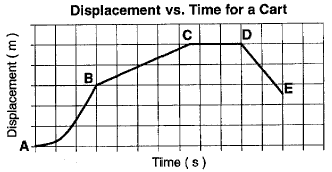# Let’s Get Physics-l: Graphs of Motion

When plotting graphs of motion, it will most likely be one of two graphs, a displacement-time graph or a velocity-time graph. From these graph, you can interpret several different things about an objects motion.On a displacement-time graph, we know that:

• Straight diagonal lines, such as the ones BC and DE, indicate that the object is travelling at a constant velocity.
• A velocity can be calculated using: Change in displacement/time taken or by calculating the gradient of the line (The same thing here)
• Flat or horizontal lines, such as CD, indicate that the object is at rest/ stationary so has no velocity.
• Curves, such as AB, indicate a non-uniform velocity.

o   A curve with an increasing positive gradient shows the object is accelerating.

o   A curve with a decreasing positive gradient show that the object is decelerating.

When interpreting the displacement-time graphs, keep these rules in mind and you should be able to calculate a number of different values from it.On a velocity-time graph, we know that:

• A diagonal line with a positive gradient indicates a constant acceleration
• A diagonal line with a negative gradient indicates a constant deceleration
• A curve indicates non uniform change in velocity
• Acceleration can be obtained by calculating the gradient of the line.
• Area below the line on a velocity time graph is equal to its displacement

Summary

• Displacement-time graph
• Straight Diagonal line – constant velocity
• Horizontal line – stationary
• Curve – Acceleration/ Deceleration
• Velocity – can be found by calculating the gradient
• Velocity-time graph
• Straight diagonal line – constant change in velocity
• Horizontal line – constant velocity
• Curve – non-uniform change in acceleration
• Area below line = Displacement
• Acceleration – equal to gradient

Not the most interesting part of kinematics but no less important when collecting and plotting data relating to kinematics. As always, if you have any thoughts, questions, feedback or suggestions please leave them in the comments.